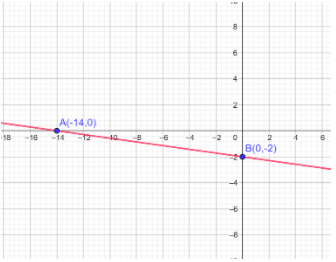How do you graph using the intercepts for $- x - 7y = 14$Verified
120.3k+ views
Hint: First we find the axis intercept values in the given equation. Also, when it is either $x$ or $y$ values are $0$. Then we have to plot the intercepts for the given equation in the graph. Finally we get the required solution.

Complete step-by-step solution:
It is given that the question we have to find the $x -$ intercept and $y -$ intercept for the straight line: $- x - 7y = 14$
Before we start solving this question, let us see what the $x -$ intercept and the $y -$ intercept which is also known as horizontal intercept and vertical intercept by putting $x = 0{\text{, y = 0 }}$ for each one respectively.
Our equation is linear function; the two intercepts can be calculated as follows:
Substituting $x = 0$ in the given equation ie., $- x - 7y = 14$
$\Rightarrow - (0) - 7y = 14$
On rewriting we get,
$\Rightarrow - 7y = 14$
On dividing $- 7$ on both sides we get,
$\Rightarrow y = \dfrac{{14}}{{ - 7}}$
Let us divide the term and we get,
$\Rightarrow y = - 2$
Hence we get the $x -$ intercept value.
$\therefore $$x - Intercept is y = - 2 Also, we need to find y - intercept. Now we are substitute, y = 0 in the given equation, \Rightarrow - x - 7y = 14 On putting the value of zero in place of y. \Rightarrow - x - 7(0) = 14 Multiplying 7 by 0 we get \Rightarrow - x - 0 = 14 By using the elementary operation, we get \Rightarrow - x = 14 Dividing the whole number 14 by ( - 1), we get \Rightarrow x = - 14 \therefore y - Intercept is x = - 14. Hence, we get \therefore$$x -$ Intercept is $y = - 2$
$\therefore y -$ Intercept is $x = - 14$.Note: $x -$ intercept is a point at which the graph intersects $x - axis$. That means we always have $y$ equal to zero Similarly we can say that for the $y -$intercept is a point at which the graph intersects $y - axis.$ That means we always have $x$ equal to zero in the coordinate system.
We have to know about the concept of $x -$ intercept and $y -$ intercept for a straight line, satisfying the condition of $x = 0{\text{ and y = 0}}$ in the equation of the given line.